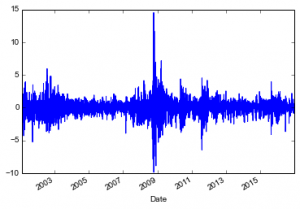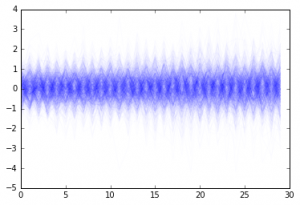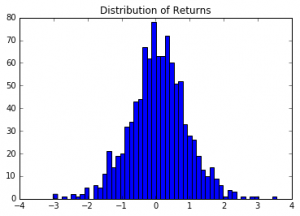Okay so I am continuing my series of posts on time-series analysis in python. So far I have covered ARIMA models, ARIMAX models, and we also looked at SARIMA models. These posts have all dealt with a similar subject. Namely, how to make a time-series be stationary in the sense that it doesn’t  have a mean dependent on time. We do this by differencing the time series, and when I say that I mean that generally to mean partial differencing and partial differencing reduction. The point is get rid of time dependence in the residual, and you can introduce that by over-differencing, and under-differencing, so ARIMA type models fix this through AR, and MA terms.

This post is a little bit different. In this post, we want to remove the dependence of the error variance on time in order to get a better model. This is where my brain starts to push back on the need for a model like this. This would mean that my model is heteroscadastic, but not necessarily unbiased. If the expected value of my errors is zero, then I should get a solid unbiased estimate slap some robust standard errors on it, and there really is no need for this model. Okay, we’re done here, pack it up everyone, we don’t need GARCH, ARCH or whatever this thing is. Let’s go home.

“What about simulating the value-at-risk from fraud? So that we can guess at how much ” the annoying part of my brain in the back shouts out. Well, I guess we could monte-carlo something with a distribution, but the volatility of the amount of money that we lose doesn’t appear to be normally distributed, but it does appear stationary. It looks like we have periods where the volatility in losses appears to be high, and then it dips down to low volatility periods. So we need some way to cluster that volatility. It looks like we need some sort of autoregressive process for volatility.

So in this post here’s what we’re going to do:

• Run a GARCH model
• Simulate the GARCH process
• Use that simulation to determine value at risk

## The Data

Okay, so our data is going to come from yahoo finance. Specifically, we’ll be looking at the S&P 500 daily returns. This data presents a very useful case study for GARCH models. Here’s the reason: The stock market tends to be pretty clumpy. And what I mean by that is that days with a lot of volatility seem to cluster together. We’ll use this to assign a value at risk to the stock market. This is a useful method, because you can say that five days from now, I know that there will be a 5% probability that I will lose more than \$1000 in the stock market or some similar value.

This is directly analogous to the fraud loss situation which I have described above. For example, we might be interested to know, what is the maximum loss that I expect to lose in the next 30 days due to fraud losses given 5% probability that my loss will be no greater. Essentially, that is the same question, as how much am I going to lose in the stock market.

So the question is, how can we obtain this data?

Well here is some code that you will need to run to start things off.

```pip install arch
```

We’ll need these packages to get the data and analyze it how we wish. Here is the data to pull the data from the internet and plot it.

```import datetime as dt
import sys

import numpy as np
import pandas as pd
import matplotlib.pyplot as plt

start = dt.datetime(2000,1,1)
end = dt.datetime(2017,1,1)
returns = 100 * sp500['Close'].pct_change().dropna()
returns.plot()
plt.show()
```

You’ll see this plot when you run this piece of code. This is what the daily returns to the S&P 500 looks like over the last 16 years.We can fit a GARCH model to this data by calling the arch package that we downloaded earlier.

```model=arch_model(returns, vol='Garch', p=1, o=0, q=1, dist='Normal')
results=model.fit()
print(results.summary())
```

This is the result that you will see when you run this code:

```Iteration:      1,   Func. Count:      6,   Neg. LLF: 5564.386282482588
Iteration:      2,   Func. Count:     16,   Neg. LLF: 5561.0681556667905
Iteration:      3,   Func. Count:     24,   Neg. LLF: 5555.458222545627
Iteration:      4,   Func. Count:     32,   Neg. LLF: 5555.246498193487
Iteration:      5,   Func. Count:     39,   Neg. LLF: 5553.738539082352
Iteration:      6,   Func. Count:     46,   Neg. LLF: 5553.06213674125
Iteration:      7,   Func. Count:     53,   Neg. LLF: 5552.394215867007
Iteration:      8,   Func. Count:     59,   Neg. LLF: 5552.16897836462
Iteration:      9,   Func. Count:     65,   Neg. LLF: 5552.15570248162
Iteration:     10,   Func. Count:     71,   Neg. LLF: 5552.155529022542
Optimization terminated successfully.    (Exit mode 0)
Current function value: 5552.155528261585
Iterations: 10
Function evaluations: 72
Constant Mean - GARCH Model Results
==============================================================================
Dep. Variable:                  Close   R-squared:                      -0.001
Mean Model:             Constant Mean   Adj. R-squared:                 -0.001
Vol Model:                      GARCH   Log-Likelihood:               -5552.16
Distribution:                  Normal   AIC:                           11112.3
Method:            Maximum Likelihood   BIC:                           11137.5
No. Observations:                 3999
Date:                Wed, Jul 05 2017   Df Residuals:                     3995
Time:                        09:05:39   Df Model:                            4
Mean Model
============================================================================
coef    std err          t      P>|t|      95.0% Conf. Int.
----------------------------------------------------------------------------
mu             0.0530  1.308e-02      4.056  4.999e-05 [2.741e-02,7.869e-02]
Volatility Model
============================================================================
coef    std err          t      P>|t|      95.0% Conf. Int.
----------------------------------------------------------------------------
omega          0.0227  5.555e-03      4.079  4.526e-05 [1.177e-02,3.354e-02]
alpha       0.1028  1.281e-02      8.023  1.035e-15   [7.768e-02,  0.128]
beta        0.8781  1.351e-02     65.006      0.000     [  0.852,  0.905]
============================================================================

Covariance estimator: robust
```

So at this point we can see a couple of things in the regression output. The beta coefficient is very close to 1 but a little bit lower. We would expect this. Basically, this is our MA term. If it was greater than 1 we would have a big problem. Essentially, when beta is bigger than 1, a small shock will grow and get louder and louder over time. In the mean this isn’t really a big deal. But in the variance of an estimator, we would have a huge problem. The variance would spiral to ever greater levels until, boom, it we hit infinite variance. Then regression would be impossible. So in terms of volatility of our model we want beta to be less than 1 in absolute value, but you can’t just take this term in isolation. Because the AR term has a similar effect, but most of the persistence should be captured in this term.

omega is the baseline variance for the model. You can think of omega as what the variance would be if information about past variances were not being passed to the model. So the square-root of omega would be the standard deviation in returns. This coefficient suggests that the standard deviation of returns should be around 15% per day. That is fairly volatile, because it implies that returns should be around 0 every day but plus or minus about 29% returns are possible. An interesting thing seems to be that we never ever see a return of anywhere near 29% in the data. Why? Because of the autocorrelation in the series, it serves to dampen the volatility, and keep the relatively low volatility days clumped together.

Finally, the alpha term tells us how much the previous period’s volatility to add to today’s volatility. It turns out that we shouldn’t expect too much clumping to be going on. According to this only about 10% of the previous days volatility will be carried over into the next day. Adding this with the beta coefficient gives a number of about 98%. If it were 100% we would have a random walk, but we see that we don’t quite have a random walk model. And it takes a really long time for a volatility regime to dissipate. This means that if we have a period of low volatility it will persist for quite some time. This helps to explain why we never see those 22% daily returns.

We expect that our returns will be about 5.3% per day as indicated by the mu coefficient.

Okay, so what is our value at risk 30 days from now. To do that we need to simulate the process into the future. We can do that with the following code:

```lines = plt.plot(sims.values[-1,:,:].T, color='blue', alpha=0.01)
lines.set_label('Simulated paths')
plt.show()
```

The output let’s us preview the simulation results. Darker regions are more likely, lighter regions are less likely paths. It’s interesting because we can see that there are definitely days that are expected to have low volatility and days that we expect higher volatility.But we aren’t so concerned, for this example, with the path that we took to get to the end of the road. We just want to know what the end of the 30 day period is going to look like. So if you imagine that we are looking down on the above figure, the darker colors would represent taller objects, and the lighter ones would be shorter. So swinging around at a 90 degree angle, we would be looking at a histogram. So let’s take a look at the histogram for the last day on this plot.

Here’s the code:

```print(np.percentile(sims.values[-1,:,-1].T,5))
plt.hist(sims.values[-1, :,-1],bins=50)
plt.title('Distribution of Returns')
plt.show()
```

We printed the 5th percentile, as well. We can interpret the amount that gives us a 5% chance of having a loss greater than this amount. So we are expecting a loss of about 138% or greater with 5% probability. And then you can do all kinds of interesting stuff. Here is the histogram of returns.And here is the complete code to reproduce everything that we have done today, or you can download it directly from github here:

```import datetime as dt
import sys

import numpy as np
import pandas as pd
import matplotlib.pyplot as plt
from arch import arch_model

start = dt.datetime(2000,1,1)
end = dt.datetime(2017,1,1)
returns = 100 * sp500['Close'].pct_change().dropna()
returns.plot()
plt.show()

model=arch_model(returns, vol='Garch', p=1, o=0, q=1, dist='Normal')
results=model.fit()
print(results.summary())

forecasts = results.forecast(horizon=30, method='simulation', simulations=1000)
sims = forecasts.simulations

lines = plt.plot(sims.values[-1,:,:].T, color='blue', alpha=0.01)
lines.set_label('Simulated paths')
plt.show()

print(np.percentile(sims.values[-1,:,-1].T,5))
plt.hist(sims.values[-1, :,-1],bins=50)
plt.title('Distribution of Returns')
plt.show()
```
Uncategorized

## 8 thoughts on “GARCH Models in Python”

1.Oliver says:

Hi,

When retrieving the simulations from the model, i encounter a MemoryError.

I am using a 365 day horizon, and the model is fitted on a lengthy time series.

Is there a way to extract simulations is a way that avoids this problem? I can’t find anything after an extensive search

1.Ryan Barnes says:

Hey Oliver,

A memory error usually means that you ran out of RAM on your machine. This means you have 3 options.

1) Simulate a smaller number of observations.
3) Move the analysis to AWS or some other cloud service.

I would personally do the first if you can get away with it, maybe reframe the problem to work with weekly data instead of daily. If you have to do 365 days, then go with option 3 since you will learn to do things remotely. That is a valuable skill in itself.

Good luck!

2.Dmitry says:

I had the same problem, although there were some 5 GB of free RAM. I ran the script in a 64-bit python and it helped! I hope it help a reader as well!

in the meantime the line
does not work

###########
or
###########

for those behind proxy:
###########
import requests
proxies = {‘http’: ‘http://…:80 or whatever’, ‘https’: ‘https://…:80 or whatever’}
requests.urllib3.ProxyManager(‘http://…:80 or whatever’,’https://…:80 or whatever’) # not sure if this line is really necessary
session = requests.Session()
session.proxies = proxies
sp500 = web.DataReader(‘SPY’,data_source=’iex’, session = session,start=start)
###########

1.trismegistos says:

iex needs API key.
use yahoo as of 2021.

2.birone says:

Thanks for this example of using using GARCH in Python – I don’t think there are many online!

“We expect that our returns will be about 5.3% per day as indicated by the mu coefficient.”
Isn’t it 0.053%? The return series is already expressed as a pct change, & 5.3% per day would mean an average annual return of ~1.5 x 10^8 (I wish 🙂

I think this also means the SD of returns is 0.15%, so a 0.9% return would be a 6*sigma (p=2 * 10^-9) event without the GARCH part of the error. (ie alpha & beta are the reason the series is so much more volatile than the mean SD would imply.)

“the alpha term tells us how much the previous period’s volatility to add to today’s volatility.”
I found the explanation of Beta – having forgotten what the MA term was – so I looked up GARCH* & it seems alpha is the coefficient on the error^2 & it’s beta that’s the coefficient on vol (both in t-1). (Perhaps there are different notations?)

1.Ryan Barnes says:

I think that you are right as rain. That’s what I get for writing it up super fast and posting it pretty late. Thanks for the heads up.

3.Farbod says:

where is sim dataframe coming from? I am no seeing where it get introduced.

1.Ryan Barnes says:

It gets defined in this line of code:

sims = forecasts.simulations

Before that line the sims dataframe does not exist.
I hope that helps!